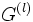Derived series

This article describes a subgroup series-defining function arising by iterating the subgroup-defining function: commutator subgroup
View other subgroup series-defining functions

Definition

Symbol-free definition

The derived series or commutator series of a group is defined as follows:

• The zeroth member is the group itself.
• The member indexed by a successor ordinal is the commutator subgroup of its predecessor.
• The member indexed by a limit ordinal is the intersection of all previous members.

The intersection of all members of the derived series of a group equals its perfect core.

Definition with symbols

The derived series of a group$G$ indexed$G^{(\alpha)}$, is defined as follows:

•$G^{(0)} = G$.
•$G^{(\alpha + 1)} = [G^\alpha,G^\alpha]$.
•$G^{(\gamma)} = \bigcap_{\alpha < \gamma} G_\alpha$ for any limit ordinal$\gamma$.

Often the term is used to refer only to the finite part of the series:$G^{(0)} = G \ge G^{(1)} = [G,G] \ge G^{(2)} = [[G,G],[G,G]] \ge \dots$.

The intersection of all members of the derived series of a group equals its perfect core.

For a solvable group

A group is solvable if and only if its derived series terminates in finitely many steps at the identity element. The smallest$l$ for which$G^{(l)}$ is the trivial group is termed the solvable length of the derived series. The derived series is the fastest descending series with the property that all successive quotients are Abelian. Note that there is no single series that serves the role of the fastest ascending series with all successive quotients Abelian.

Further information: Derived series is fastest descending series with Abelian quotients

Facts

Subgroup properties satisfied by members

The derived series is a strongly fully invariant series and hence a strongly characteristic series: every smaller member of the series is a fully invariant subgroup in every bigger (earlier) member, and hence also a characteristic subgroup in every bigger (earlier) member. Each member is a fully characteristic subgroup; in fact, each member is an image-closed fully invariant subgroup. The finite members are also verbal subgroups.

Related group properties

• Perfect group: A group whose derived series terminates at the group itself: it equals its commutator subgroup.
• Solvable group: A group whose derived series terminates in finitely many steps at the trivial subgroup.
• Hypoabelian group: A group whose transfinite derived series terminates at the trivial subgroup. Equivalently, it is a group whose perfect core is trivial.
• Residually solvable group: A group such that the intersection of all finite members of its derived series is the trivial subgroup. Equivalently, for every element of the group, there is a normal subgroup of finite index not containing that element such that the quotient by that subgroup is a solvable group.